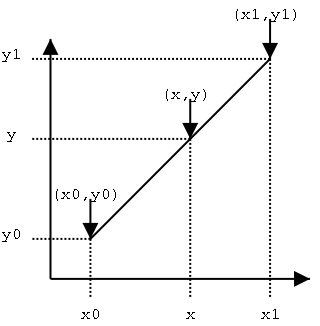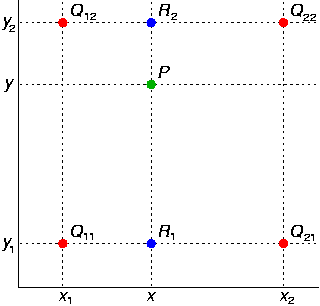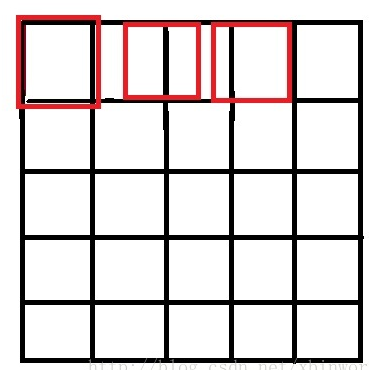## 0. 前言

• 目标：自己写代码实现双线性插值，并能讲出道理来。
• 参考资料：

## 1. 线性插值

• 维基百科配图如下：
• 加上一点自己的理解：不能直接用于图像插值，毕竟这个只能补全一条对角线上的值。
•• 要解决的问题：
• 已知 (x0, y0)(x1, y1)，以及 (x,y)中的一个值。
• 即使用 x, x0, y0, x1, y1 计算 y 或使用 y, x0, y0, x1, y1 计算 x
• 计算公式很容易，将上面的参数带入下面公式即可：$$\frac{y-y_0}{x-x_0} = \frac{y_1-y_0}{x_1-x_0}$$
• 应用举例：
• 假设一个表格列出了一个国家 1970年、1980年、1990年以及 2000年的人口，那么如果需要估计 1994年的人口的话，线性插值就是一种简便的方法。
• 可以通过三步线性插值完成一次双线性插值运算。

## 2. 双线性插值

### 2.1. 理论介绍

• 维基百科配图如下：
• 加上一点自己的理解：
• 不仅要知道x1, x2, y1, y2, x, y的取值，还需要知道 Q11, Q12, Q21, Q22的取值。
• 先经过线性插值计算R1, R2的取值，再通过线性插值计算P的取值。
• 即，双线性插值是通过三次线性插值获取。
•• 要解决的问题：
• 假设像素值的函数为 f(x, y)
• 在已知 x1, x2, y1, y2 以及Q11, Q12, Q21, Q22四个位置像素值的前提下，计算$(x, y)， x \in [x_0, x_1], y \in [y_0, y1]$处的取值。
• 假设 Q11, Q12, Q21, Q22 四个位置像素值为 f(Q11), f(Q12), f(Q21), f(Q22)
• 从图像插值的角度看，x, y, x1, y1, x2, y2都是像素的坐标位置，Q11, Q12, Q21, Q22 是四个位置对应的坐标，f(Q11), f(Q12), f(Q21), f(Q22)为对应的像素值，要计算(x, y)的是像素值。
• 具体过程：
• 首先对x轴方向进行线性插值：
• $$R_1 = f(x, y_1) \approx \frac{x_2-x}{x_2-x_1}f(Q_{11}) + \frac{x_2-x}{x_2-x_1}f(Q_{21})$$
• $$R_2 = f(x, y_1) \approx \frac{x_2-x}{x_2-x_1}f(Q_{12}) + \frac{x_2-x}{x_2-x_1}f(Q_{22})$$
• 再通过 R1, R2计算线性插值，获取P的结果：
• $$f(x, y) \approx \frac{y_2-y}{y_2-y_1} R_1 + \frac{y-y_1}{y_2-y_1} R2$$
• 先从y轴、再从x轴进行线性插值的结果是一样的。

### 2.2. 具体实现介绍

• 具体实现描述：
• 假设初始图片为 img1，尺寸为h,w，像素点坐标作为记作 $(x_i, y_i)$。
• 假设目标图像 img2，图像尺寸为 hh, ww，像素点坐标记作 $(xx_i, yy_i)$。
• 双线性插值的目标是以img1作为输入，获取图像 img2
• 实现步骤：
• 找到目标图像img2中每个像素点 $(xx_i, yy_i)$对应于原始图像中的位置，记作 $(x_i’, y_i’)$
• $(x_i’, y_i’)$ 就是 2.1. 配图中点P的位置。
• 通过 ceil/floor 操作获取 Q11, Q12, Q21, Q22 对应的坐标值。
• 之后就可以通过 2.1. 中的双线性插值的具体过程获取对应点像素值。
• 实现的重点与难点：就是上述实现步骤一，获取目标图像中每个像素点对应于原始图像中的坐标。
• 根据比例获取对应坐标：
• 根据上面的假设，可以获得两个比例 $scale_h = h/hh, scale_w = w/ww$。
• 那么对于目标图像中每一个坐标 $(xx_i, yy_i)$ 就对应于原始图像中的：$x_i’ = scale_h * xx_i, y_i’ = scale_w * yy_i$
• 这样做存在的问题：实现过程中，所有坐标都是从0开始编号的，这就会导致有偏移。
• 举个例子，如果想把5*5的图像转换为3*3，那么最终得到的三个横坐标分别是 0, 5/3, 10/3，很显然，这结果偏移到左边了。这个结果，也就是很多博客中提到的配图（注意下图中的横坐标）：
•• 为了解决根据比例获取对应坐标存在的问题，cv2等库都使用了中心对齐获取坐标
• 从实现角度看很简单，将原本的转换公式 $x_i’ = scale_h * xx_i, y_i’ = scale_w * yy_i$ 稍微修改一下。
• 改成：$x_i’ = scale_h * (xx_i + 0.5) - 0.5, y_i’ = scale_w * (yy_i + 0.5) - 0.5$